Concevoir un site comme celui-ci avec WordPress.com

# Fission nucléaire et fusion nucléaire : quelle différence ? | Dossier Radioactivity

Pour consulter ce dossier, cliquez sur le lien ci-dessous.

La fission et la fusion sont deux grandes classes de transformation nucléaire provoquée, c’est à dire qu’elles ne se produisent qu’après une intervention extérieure.

Les réactions de fission résultent du bombardment d’un noyau lourd par un neutron.

Les réactions de fusion résultent de l’assemblage de noyaux légers pour donner naissance à un noyau plus lourd qu’eux-mêmes

Pour plus d’informations sur la fission et la fusion, cliquez sur le lien ci-dessous

https://www.futura-sciences.com/sciences/dossiers/physique-energie-nucleaire-a-z-126/page/5/

Avec la découverte de la radioactivité, les physiciens sont confrontés à un problème de taille : lors des réactions de désintégration, la masse des éléments produits est toujours plus faible que celle des éléments au départ. Mais où est passée cette masse manquante ?
La réponse est donnée en 1905 par le physicien EINSTEIN quand sa théorie de la relativité restreinte l’amène à formuler la formule E = m c2. Mettant ainsi en évidence, l’équivalence entre la masse m et l’énergie E.
Cette formule va véritablement révolutionner le monde et permettre le développement de l’industrie nucléaire militaire puis civile. Il recevra le prix Nobel de physique en 1921 pour ses contributions à la physique théorique, spécialement pour la loi de l’effet photoélectrique

## Frédéric and Irène Joliot Curie

Frédéric and Irène Joliot-Curie, original names (until 1926) Jean-Frédéric Joliot and Irène Curie, (respectively, born March 19, 1900, Paris, France—died Aug. 14, 1958, Arcouest; born Sept. 12, 1897, Paris—died March 17, 1956, Paris), French physical chemists, husband and wife, who were jointly awarded the 1935 Nobel Prize for Chemistry for their discovery of new radioactive isotopes prepared artificially. They were the son-in-law and daughter of Nobel Prize winners Pierre and Marie Curie.

Irène Curie from 1912 to 1914 prepared for her baccalauréat at the Collège Sévigné and in 1918 became her mother’s assistant at the Institut du Radium of the University of Paris. In 1925 she presented her doctoral thesis on the alpha rays of polonium. In the same year she met Frédéric Joliot in her mother’s laboratory; she was to find in him a mate who shared her interest in science, sports, humanism, and the arts.Marie CurieMarie Curie (far right) and her daughter Irène (second from right) posing with their pupils from the American Expeditionary Forces at the Institut du Radium, Paris, 1919.© Photos.com/Jupiterimages

Joliot simultaneously pursued new studies to obtain his licence ès sciences in 1927, taught at the École d’Électricité Industrielle Charliat in order to augment his finances, and learned laboratory techniques under the guidance of Irène Curie. Beginning in 1928 they signed their scientific work jointly.Get a Britannica Premium subscription and gain access to exclusive content.Subscribe Now

In the course of their researches they bombarded boron, aluminum, and magnesium with alpha particles; and they obtained radioactive isotopes of elements not ordinarily radioactive, namely, nitrogenphosphorus, and aluminum. These discoveries revealed the possibility of using artificially produced radioactive isotopes to follow chemical changes and physiological processes, and such applications were soon successful; the absorption of radioiodine by the thyroid gland was detected, and the course of radiophosphorus (in the form of phosphates) was traced in the metabolism of the organism. The production of these unstable atomic nuclei afforded further means for the observation of changes in the atom as these nuclei broke down. The Joliot-Curies observed also the production of neutrons and positive electrons in the changes that they studied; and their discovery of artificial radioactive isotopes constituted an important step toward the solution of the problem of releasing the energy of the atom, since the method of Enrico Fermi, using neutrons instead of alpha particles for the bombardments which led to the fission of uranium, was an extension of the method developed by the Joliot-Curies for producing radioelements artificially.

In 1935 Frédéric and Irène Joliot-Curie were awarded the Nobel Prize for Chemistry for the synthesis of new radioactive isotopes. The Joliot-Curies then moved into a home at the edge of the Parc de Sceaux. They left it only for visits to their house in Brittany at Pointe de l’Arcouest, where university families had been meeting together since the time of Marie Curie. And, for the sake of Irène’s lungs, they visited the mountains of Courchevel during the 1950s.

Frédéric, appointed professor at the Collège de France in 1937, devoted a part of his activities to preparing new sources of radiation. He then supervised the construction of electrostatic accelerators at Arcueil-Cachan and at Ivry and a cyclotron of seven million electron volts at the Collège de France, the second (after the Soviet Union) installation of equipment of this type in Europe.

Irène then devoted her time largely to the upbringing of their children, Hélène and Pierre. But both she and Frédéric had a lofty idea of their human and social responsibilities. They had joined the Socialist Party in 1934 and the Comité de Vigilance des Intellectuels Antifascistes (Vigilance Committee of Anti-Fascist Intellectuals) in 1935. They also took a stand in 1936 on the side of Republican Spain. Irène was one of three women to participate in the Popular Front government of 1936. As undersecretary of state for scientific research, she helped to lay the foundations, with Jean Perrin, for what would later become the Centre National de la Recherche Scientifique (National Centre for Scientific Research).

Pierre and Marie Curie had decided to publish everything. This was also the attitude adopted by the Joliot-Curies for the discovery of artificial radioactive isotopes. But anxiety resulting from the rise of Nazism and the awareness of the dangers that could result from the application of chain reactions led them to cease publication. On Oct. 30, 1939, they recorded the principle of nuclear reactors in a sealed envelope, which they deposited at the Académie des Sciences; it remained secret until 1949. Frédéric chose to remain in occupied France with his family and to make certain that the Germans who came into his laboratory could not use his work or his equipment, whose removal to Germany he prevented. The Joliot-Curies continued their research, notably in biology; after 1939, Frédéric demonstrated, with Antoine Lacassagne, the use of radioactive iodine as a tracer in the thyroid gland. He became a member of the Académie de Médecine in 1943.

But the struggle against the occupying forces began to require more and more of his attention. In November of 1940 he denounced the imprisonment of Paul Langevin. In June of 1941 he took part in the founding of the National Front Committee, of which he became the president. In the spring of 1942, after the execution by the Nazis of the theoretical physicist J. Solomon, Frédéric joined the French Communist Party, of which in 1956 he became a member of the central committee. He created the Société d’Études des Applications des Radio-éléments Artificiels, an industrial company that gave work certificates to scientists and thus prevented their being sent to Germany. In May 1944, Irène and their children took refuge in Switzerland, and Frédéric lived in Paris under the name of Jean-Pierre Gaumont. His laboratory at the Collège de France, at which he organized the production of explosives, served as an arsenal during the battle for the liberation of Paris. In recognition, he was designated a commander of the Legion of Honour with a military title and was decorated with the Croix de Guerre.

In France, after the liberation in 1944, Frédéric was elected to the Académie des Sciences and was entrusted with the position of director of the Centre National de la Recherche Scientifique.

Then, in 1945 General de Gaulle authorized Frédéric and the minister of armaments to create the Commissariat à l’Energie Atomique to ensure for France the applications of the discoveries made in 1939. Irène devoted her scientific experience and her abilities as an administrator to the acquisition of raw materials, the prospecting for uranium, and the construction of detection installations. In 1946 she was also appointed director of the Institut du Radium. Frédéric’s efforts culminated in the deployment, on Dec. 15, 1948, of ZOE (zéro, oxyde d’uranium, eau lourde), the first French nuclear reactor, which, though only moderately powerful, marked the end of the Anglo-Saxon monopoly. In April 1950, however, during the climax of the cold war and anticommunism, Prime Minister Georges Bidault removed him without explanation from his position as high commissioner, and a few months later Irène was also deprived of her position as commissioner in the Commissariat à l’Energie Atomique. They devoted themselves henceforth to their own laboratory work, to teaching, and to various peace movements. Irène wrote the entry on polonium for the 1949 printing of the 14th edition of the Encyclopædia Britannica.

During the 1950s, following several operations, Irène’s health began to decline. In May of 1953 Frédéric had the first attack of hepatitis from which he was to suffer for five years, with a serious relapse in 1955. In 1955 Irène drew up plans for the new nuclear physics laboratories at the Université d’Orsay, south of Paris, where teams of scientists could work with large particle accelerators under conditions less cramped than in the Parisian laboratories. Early in 1956 Irène was sent into the mountains, but her condition did not improve. Wasted away by leukemia as her mother had been, she again entered the Curie Hospital, where she died in 1956.

Ill and knowing that his days were also numbered, Frédéric decided to carry on Irène’s unfinished work. In September 1956 he accepted the position of professor at the University of Paris left vacant by Irène, at the same time occupying his own chair at the Collège de France. He successfully completed the establishment of the Orsay laboratories and saw the start of research there in 1958.

radioactivity, property exhibited by certain types of matter of emitting energy and subatomic particles spontaneously. It is, in essence, an attribute of individual atomic nuclei.

An unstable nucleus will decompose spontaneously, or decay, into a more stable configuration but will do so only in a few specific ways by emitting certain particles or certain forms of electromagnetic energy. Radioactive decay is a property of several naturally occurring elements as well as of artificially produced isotopes of the elements. The rate at which a radioactive element decays is expressed in terms of its half-life; i.e., the time required for one-half of any given quantity of the isotope to decay. Half-lives range from more than 1024 years for some nuclei to less than 10−23 second (see below Rates of radioactive transitions). The product of a radioactive decay process—called the daughter of the parent isotope—may itself be unstable, in which case it, too, will decay. The process continues until a stable nuclide has been formed.

## The nature of radioactive emissions

The emissions of the most common forms of spontaneous radioactive decay are the alpha (α) particle, the beta (β) particle, the gamma (γ) ray, and the neutrino. The alpha particle is actually the nucleus of a helium-4 atom, with two positive charges 4/2He. Such charged atoms are called ions. The neutral helium atom has two electrons outside its nucleus balancing these two charges. Beta particles may be negatively charged (beta minus, symbol e), or positively charged (beta plus, symbol e+). The beta minus [β] particle is actually an electron created in the nucleus during beta decay without any relationship to the orbital electron cloud of the atom. The beta plus particle, also called the positron, is the antiparticle of the electron; when brought together, two such particles will mutually annihilate each other. Gamma rays are electromagnetic radiations such as radio waves, light, and X-rays. Beta radioactivity also produces the neutrino and antineutrino, particles that have no charge and very little mass, symbolized by ν and ν, respectively.

In the less common forms of radioactivity, fission fragments, neutrons, or protons may be emitted. Fission fragments are themselves complex nuclei with usually between one-third and two-thirds the charge Z and mass A of the parent nucleus. Neutrons and protons are, of course, the basic building blocks of complex nuclei, having approximately unit mass on the atomic scale and having zero charge or unit positive charge, respectively. The neutron cannot long exist in the free state. It is rapidly captured by nuclei in matter; otherwise, in free space it will undergo beta-minus decay to a proton, an electron, and an antineutrino with a half-life of 12.8 minutes. The proton is the nucleus of ordinary hydrogen and is stable.Get a Britannica Premium subscription and gain access to exclusive content.Subscribe Now

The early work on natural radioactivity associated with uranium and thorium ores identified two distinct types of radioactivity: alpha and beta decay.

## Alpha decay

In alpha decay, an energetic helium ion (alpha particle) is ejected, leaving a daughter nucleus of atomic number two less than the parent and of atomic mass number four less than the parent. An example is the decay (symbolized by an arrow) of the abundant isotope of uranium238U, to a thorium daughter plus an alpha particle:Given for this and subsequent reactions are the energy released (Q) in millions of electron volts (MeV) and the half-life (t1⁄2). It should be noted that in alpha decays the charges, or number of protons, shown in subscript are in balance on both sides of the arrow, as are the atomic masses, shown in superscript.

## Beta-minus decay

In beta-minus decay, an energetic negative electron is emitted, producing a daughter nucleus of one higher atomic number and the same mass number. An example is the decay of the uranium daughter product thorium-234 into protactinium-234:In the above reaction for beta decay, ν represents the antineutrino. Here, the number of protons is increased by one in the reaction, but the total charge remains the same, because an electron, with negative charge, is also created.

# Gamma decay

A third type of radiation, gamma radiation, usually accompanies alpha or beta decay. Gamma rays are photons and are without rest mass or charge. Alpha or beta decay may simply proceed directly to the ground (lowest energy) state of the daughter nucleus without gamma emission, but the decay may also proceed wholly or partly to higher energy states (excited states) of the daughter. In the latter case, gamma emission may occur as the excited states transform to lower energy states of the same nucleus. (Alternatively to gamma emission, an excited nucleus may transform to a lower energy state by ejecting an electron from the cloud surrounding the nucleus. This orbital electron ejection is known as internal conversion and gives rise to an energetic electron and often an X-ray as the atomic cloud fills in the empty orbital of the ejected electron. The ratio of internal conversion to the alternative gamma emission is called the internal-conversion coefficient.)

## Isomeric transitions

There is a wide range of rates of half-lives for the gamma-emission process. Usually dipole transitions (see below Gamma transition), in which the gamma ray carries off one ℏ unit of angular momentum, are fast, less than nanoseconds (one nanosecond equals 10−9 second). The law of conservation of angular momentum requires that the sum of angular momenta of the radiation and daughter nucleus is equal to the angular momentum (spin) of the parent. If the spins of initial and final states differ by more than one, dipole radiation is forbidden, and gamma emission must proceed more slowly by a higher multipole (quadrupole, octupole, etc.) gamma transition. If the gamma-emission half-life exceeds about one nanosecond, the excited nucleus is said to be in a metastable, or isomeric, state (the names for a long-lived excited state), and it is customary to classify the decay as another type of radioactivity, an isomeric transition. An example of isomerism is found in the protactinium-234 nucleus of the uranium-238 decay chain:The letter m following the mass number stands for metastable and indicates a nuclear isomer.

## Beta-plus decay

During the 1930s new types of radioactivity were found among the artificial products of nuclear reactions: beta-plus decay, or positron emission, and electron capture. In beta-plus decay an energetic positron is created and emitted, along with a neutrino, and the nucleus transforms to a daughter, lower by one in atomic number and the same in mass number. For instance, carbon-11 (Z = 6) decays to boron-11 (Z = 5), plus one positron and one neutrino:## Electron capture

Electron capture (EC) is a process in which decay follows the capture by the nucleus of an orbital electron. It is similar to positron decay in that the nucleus transforms to a daughter of one lower atomic number. It differs in that an orbital electron from the cloud is captured by the nucleus with subsequent emission of an atomic X-ray as the orbital vacancy is filled by an electron from the cloud about the nucleus. An example is the nucleus of beryllium-7 capturing one of its inner electrons to give lithium-7:The main features of radioactive decay of a nuclear species are often displayed in a decay scheme. Figure 1 shows the decay scheme of beryllium-7. Indicated are the half-life of the parent and that of the excited daughter state, as well as its energy 0.4774 MeV. The spins and parities of all three states are provided on the upper left-hand side of the level. The multipolarity of the gamma ray (magnetic dipole, M1, plus 0.005 percent electric quadrupole, E2) is indicated above the vertical arrow symbolizing the gamma transition. The slanted arrows symbolize the electron-capture decay with labels giving the percentage of decay directly to ground state (89.7 percent) and the percentage of EC decay going via the excited state (10.3 percent). The boldface numbers following the percentages are so-called log ft values, to be encountered below in connection with beta-decay rates. The overall energy release, QEC, is indicated below. The QEC is necessarily a calculated value because there is no general practical means of measuring the neutrino energies accompanying EC decay. With a few electron-capturing nuclides, it has been possible to measure directly the decay energy by measurement of a rare process called inner bremsstrahlung (braking radiation). In this process the energy release is shared between the neutrino and a gamma ray. The measured distribution of gamma-ray energies indicates the total energy release. Usually there is so much ordinary gamma radiation with radioactive decay that the inner bremsstrahlung is unobservable.decay of beryllium-7Figure 1: Radioactive decay of beryllium-7 to lithium-7 by electron capture (EC; see text).Encyclopædia Britannica, Inc.

## Spontaneous fission

Yet another type of radioactivity is spontaneous fission. In this process the nucleus splits into two fragment nuclei of roughly half the mass of the parent. This process is only barely detectable in competition with the more prevalent alpha decay for uranium, but for some of the heaviest artificial nuclei, such as fermium-256, spontaneous fission becomes the predominant mode of radioactive decay. Kinetic-energy releases from 150 to 200 MeV may occur as the fragments are accelerated apart by the large electrical repulsion between their nuclear charges. The reaction is as follows:Only one of several product sets is shown. A few neutrons are always emitted in fission of this isotope, a feature essential to chain reactions. Spontaneous fission is not to be confused with induced fission, the process involved in nuclear reactors. Induced fisson is a property of uranium-235, plutonium-239, and other isotopes to undergo fission after absorption of a slow neutron. Other than the requirement of a neutron capture to initiate it, induced fission is quite similar to spontaneous fission regarding total energy release, numbers of secondary neutrons, and so on (see nuclear fission).

Proton radioactivity, discovered in 1970, is exhibited by an excited isomeric state of cobalt-53, 53mCo, 1.5 percent of which emits protons: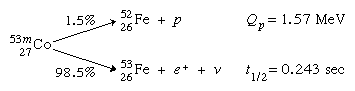## Special beta-decay processes

In addition to the above types of radioactivity, there is a special class of rare beta-decay processes that gives rise to heavy-particle emission. In these processes the beta decay partly goes to a high excited state of the daughter nucleus, and this state rapidly emits a heavy particle.

One such process is beta-delayed neutron emission, which is exemplified by the following reaction: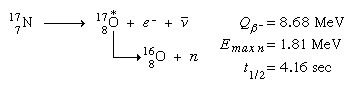(Note: the asterisk denotes the short-lived intermediate excited states of oxygen-17, and Emax n denotes the maximum energy observed for emitted neutrons.) There is a small production of delayed neutron emitters following nuclear fission, and these radioactivities are especially important in providing a reasonable response time to allow control of nuclear fission reactors by mechanically moved control rods.

Among the positron emitters in the light-element region, a number beta decay partly to excited states that are unstable with respect to emission of an alpha particle. Thus, these species exhibit alpha radiation with the half-life of the beta emission. Both the positron decay from boron-8 and electron decay from lithium-8 are beta-delayed alpha emission, because ground as well as excited states of beryllium-8 are unstable with respect to breakup into two alpha particles. Another example, sodium-20 (20Na) to give successively neon-20 (20Ne; the asterisk again indicating the short-lived intermediate state) and finally oxygen-16 is listed below: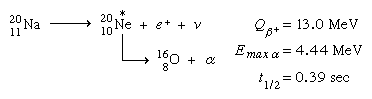In a few cases, positron decay leads to an excited nuclear state not able to bind a proton. In these cases, proton radiation appears with the half-life of the beta transition. The combination of high positron-decay energy and low proton-binding energy in the daughter ground state is required. In the example given below, tellurium-111 (111Te) yields antimony-111 (111Sb) and then tin-110 (110Sn) successively: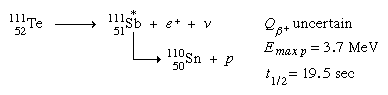In 1980 A. Sandulescu, D.N. Poenaru, and W. Greiner described calculations indicating the possibility of a new type of decay of heavy nuclei intermediate between alpha decay and spontaneous fission. The first observation of heavy-ion radioactivity was that of a 30-MeV, carbon-14 emission from radium-223 by H.J. Rose and G.A. Jones in 1984. The ratio of carbon-14 decay to alpha decay is about 5 × 10−10. Observations also have been made of carbon-14 from radium-222, radium-224, and radium-226, as well as neon-24 from thorium-230, protactinium-231, and uranium-232. Such heavy-ion radioactivity, like alpha decay and spontaneous fission, involves quantum-mechanical tunneling through the potential-energy barrier. Shell effects play a major role in this phenomenon, and in all cases observed to date the heavy partner of carbon-14 or neon-24 is close to doubly magic lead-208 (see below Nuclear models).

Some species of radioactivity occur naturally on Earth. A few species have half-lives comparable to the age of the elements (about 6 × 109 years), so that they have not decayed away after their formation in stars. Notable among these are uranium-238, uranium-235, and thorium-232. Also, there is potassium-40, the chief source of irradiation of the body through its presence in potassium of tissue. Of lesser significance are the beta emitters vanadium-50, rubidium-87, indium-115, tellurium-123, lanthanum-138, lutetium-176, and rhenium-187, and the alpha emitters cerium-142, neodymium-144, samarium-147, gadolinium-152, dysprosium-156, hafnium-174, platinum-190, and lead-204. Besides these approximately 109-year species, there are the shorter-lived daughter activities fed by one or another of the above species; e.g., by various nuclei of the elements between lead (Z = 82) and thorium (Z = 90).

Another category of natural radioactivity includes species produced in the upper atmosphere by cosmic ray bombardment. Notable are 5,720-year carbon-14 and 12.3-year tritium (hydrogen-3), 53-day beryllium-7, and 2,700,000-year beryllium-10. Meteorites are found to contain additional small amounts of radioactivity, the result of cosmic ray bombardments during their history outside the Earth’s atmospheric shield. Activities as short-lived as 35-day argon-37 have been measured in fresh falls of meteorites. Nuclear explosions since 1945 have injected additional radioactivities into the environment, consisting of both nuclear fission products and secondary products formed by the action of neutrons from nuclear weapons on surrounding matter.

The fission products encompass most of the known beta emitters in the mass region 75–160. They are formed in varying yields, rising to maxima of about 7 percent per fission in the mass region 92–102 (light peak of the fission yield versus atomic mass curve) and 134–144 (heavy peak). Two kinds of delayed hazards caused by radioactivity are recognized. First, the general radiation level is raised by fallout settling to Earth. Protection can be provided by concrete or earth shielding until the activity has decayed to a sufficiently low level. Second, ingestion or inhalation of even low levels of certain radioactive species can pose a special hazard, depending on the half-life, nature of radiations, and chemical behaviour within the body. For a detailed discussion on the biological effects of radiation, see radiation: Biological effects of ionizing radiation.

Nuclear reactors also produce fission products but under conditions in which the activities may be contained. Containment and waste-disposal practices should keep the activities confined and eliminate the possibility of leaching into groundwaters for times that are long compared to the half-lives. A great advantage of thermonuclear fusion power over fission power, if it can be practically realized, is not only that its fuel reserves, heavy hydrogen and lithium, are vastly greater than uranium, but also that the generation of radioactive fission product wastes can be largely avoided. In this connection, it may be noted that a major source of heat in the interior of both the Earth and the Moon is provided by radioactive decay. Theories about the formation and evolution of the Earth, Moon, and other planets must take into account these large heat production sources.

Desired radioactivities other than natural activities and fission products may be produced either by irradiation of certain selected target materials by reactor neutrons or by charged particle beams or gamma ray beams of accelerators.

## Energy release in radioactive transitions

Consideration of the energy release of various radioactive transitions leads to the fundamental question of nuclear binding energies and stabilities. A much-used method of displaying nuclear-stability relationships is an isotope chart, those positions on the same horizontal row corresponding to a given proton number (Z) and those on the same vertical column to a given neutron number (N). Such a map is shown in Figure 2. The irregular bold line surrounds the region of presently known nuclei. The area encompassed by this is often referred to as the valley of stability because the chart may be considered a map of a binding energy surface, the lowest areas of which are the most stable. The most tightly bound nuclei of all are the abundant iron and nickel isotopes. Near the region of the valley containing the heaviest nuclei (largest mass number A; i.e., largest number of nucleons, N + Z), the processes of alpha decay and spontaneous fission are most prevalent; both these processes relieve the energetically unfavourable concentration of positive charge in the heavy nuclei.

Along the region that borders on the valley of stability on the upper left-hand side are the positron-emitting and electron-capturing radioactive nuclei, with the energy release and decay rates increasing the farther away the nucleus is from the stability line. Along the lower right-hand border region, beta-minus decay is the predominant process, with energy release and decay rates increasing the farther the nucleus is from the stability line.

The grid lines of the graph are at the nucleon numbers corresponding to extra stability, the “magic numbers” (see below Nuclear models). The circles labeled “deformed regions” enclose regions in which nuclei should exhibit cigar shapes; elsewhere the nuclei are spherical. Outside the dashed lines nuclei would be unbound with respect to neutron or proton loss and would be exceedingly short-lived (less than 10−19 second).

# Calculation and measurement of energy

By the method of closed energy cycles, it is possible to use measured radioactive-energy-release (Q) values for alpha and beta decay to calculate the energy release for unmeasured transitions. An illustration is provided by the cycle of four nuclei below: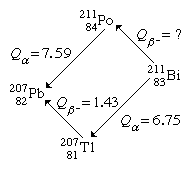In this cycle, energies from two of the alpha decays and one beta decay are measurable. The unmeasured beta-decay energy for bismuth-211, Qβ−(Bi), is readily calculated because conservation of energy requires the sum of Q values around the cycle to be zero. Thus, Qβ−(Bi) + 7.59 − 1.43 − 6.75 = 0. Solving this equation gives Qβ−(Bi) = 0.59 MeV. This calculation by closed energy cycles can be extended from stable lead-207 back up the chain of alpha and beta decays to its natural precursor uranium-235 and beyond. In this manner the nuclear binding energies of a series of nuclei can be linked together. Because alpha decay decreases the mass number A by 4, and beta decay does not change A, closed α−β-cycle calculations based on lead-207 can link up only those nuclei with mass numbers of the general type A = 4n + 3, in which n is an integer. Another, the 4n series, has as its natural precursor thorium-232 and its stable end product lead-208. Another, the 4n + 2 series, has uranium-238 as its natural precursor and lead-206 as its end product.READ MORE ON THIS TOPICatom: Radioactive decayThe nuclei of most everyday atoms are stable—that is, they do not change over time. This statement is somewhat misleading, however, because…

In early research on natural radioactivity, the classification of isotopes into the series cited above was of great significance because they were identified and studied as families. Newly discovered radioactivities were given symbols relating them to the family and order of occurrence therein. Thus, thorium-234 was known as UX1, the isomers of protactinium-234 as UX2 and UZ, uranium-234 as UII, and so forth. These original symbols and names are occasionally encountered in more recent literature but are mainly of historical interest. The remaining 4n + 1 series is not naturally occurring but comprises well-known artificial activities decaying down to stable thallium-205.

To extend the knowledge of nuclear binding energies, it is clearly necessary to make measurements to supplement the radioactive-decay energy cycles. In part, this extension can be made by measurement of Q values of artificial nuclear reactions. For example, the neutron-binding energies of the lead isotopes needed to link the energies of the four radioactive families together can be measured by determining the threshold gamma-ray energy to remove a neutron (photonuclear reaction); or the energies of incoming deuteron and outgoing proton in the reaction can be measured to provide this information.

Further extensions of nuclear-binding-energy measurements rely on precision mass spectroscopy (see spectroscopy). By ionizing, accelerating, and magnetically deflecting various nuclides, their masses can be measured with great precision. A precise measurement of the masses of atoms involved in radioactive decay is equivalent to direct measurement of the energy release in the decay process. The atomic mass of naturally occurring but radioactive potassium-40 is measured to be 39.964008 amu. Potassium-40 decays predominantly by β-emission to calcium-40, having a measured mass 39.962589. Through Einstein’s equation, energy is equal to mass (m) times velocity of light (c) squared, or E = mc2, the energy release (Q) and the mass difference, Δm, are related, the conversion factor being one amu, equal to 931.478 MeV. Thus, the excess mass of potassium-40 over calcium-40 appears as the total energy release Qβ in the radioactive decay Qβ− = (39.964008 − 39.962589) × 931.478 MeV = 1.31 MeV. The other neighbouring isobar (same mass number, different atomic number) to argon-40 is also of lower mass, 39.962384, than potassium-40. This mass difference converted to energy units gives an energy release of 1.5 MeV, this being the energy release for EC decay to argon-40. The maximum energy release for positron emission is always less than that for electron capture by twice the rest mass energy of an electron (2m0c2 = 1.022 MeV); thus, the maximum positron energy for this reaction is 1.5 − 1.02, or 0.48 MeV.

To connect alpha-decay energies and nuclear mass differences requires a precise knowledge of the alpha-particle (helium-4) atomic mass. The mass of the parent minus the sum of the masses of the decay products gives the energy release. Thus, for alpha decay of plutonium-239 to uranium-235 and helium-4 the calculation goes as follows: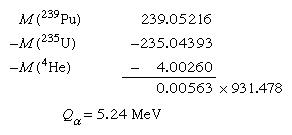By combining radioactive-decay-energy information with nuclear-reaction Q values and precision mass spectroscopy, extensive tables of nuclear masses have been prepared. From them the Q values of unmeasured reactions or decay may be calculated.

Alternative to the full mass, the atomic masses may be expressed as mass defect, symbolized by the Greek letter delta, Δ (the difference between the exact mass M and the integer A, the mass number), either in energy units or atomic mass units.

## Absolute nuclear binding energy

The absolute nuclear binding energy is the hypothetical energy release if a given nuclide were synthesized from Z separate hydrogen atoms and N (equal to A − Z) separate neutrons. An example is the calculation giving the absolute binding energy of the stablest of all nuclei, iron-56: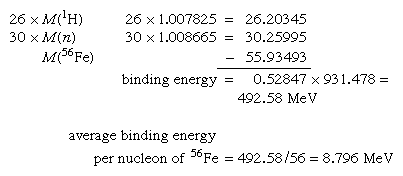A general survey of the average binding energy per nucleon (for nuclei of all elements grouped according to ascending mass) shows a maximum at iron-56 falling off gradually on both sides to about 7 MeV at helium-4 and to about 7.4 MeV for the most massive nuclei known. Most of the naturally occurring nuclei are thus not stable in an absolute nuclear sense. Nuclei heavier than iron would gain energy by degrading into nuclear products closer to iron, but it is only for the elements of greatest mass that the rates of degradation processes such as alpha decay and spontaneous fission attain observable rates. In a similar manner, nuclear energy is to be gained by fusion of most elements lighter than iron. The coulombic repulsion between nuclei, however, keeps the rates of fusion reactions unobservably low unless the nuclei are subjected to temperatures of greater than 107 K. Only in the hot cores of the Sun and other stars or in thermonuclear bombs or controlled fusion plasmas are these temperatures attained and nuclear-fusion energy released.

# Nuclear models

## The liquid-drop model

The average behaviour of the nuclear binding energy can be understood with the model of a charged liquid drop. In this model, the aggregate of nucleons has the same properties of a liquid drop, such as surface tensioncohesion, and deformation. There is a dominant attractive-binding-energy term proportional to the number of nucleons A. From this must be subtracted a surface-energy term proportional to surface area and a coulombic repulsion energy proportional to the square of the number of protons and inversely proportional to the nuclear radius. Furthermore, there is a symmetry-energy term of quantum-mechanical origin favouring equal numbers of protons and neutrons. Finally, there is a pairing term that gives slight extra binding to nuclei with even numbers of neutrons or protons.

The pairing-energy term accounts for the great rarity of odd–odd nuclei (the terms odd–odd, even–even, even–odd, and odd–even refer to the evenness or oddness of proton numberZ, and neutron number, N, respectively) that are stable against beta decay. The sole examples are deuterium, lithium-6, boron-10, and nitrogen-14. A few other odd–odd nuclei, such as potassium-40, occur in nature, but they are unstable with respect to beta decay. Furthermore, the pairing-energy term makes for the larger number of stable isotopes of even-Z elements, compared to odd-Z, and for the lack of stable isotopes altogether in element 43, technetium, and element 61, promethium.

The beta-decay energies of so-called mirror nuclei afford one means of estimating nuclear sizes. For example, the neon and fluorine nuclei, 19/10Ne9 and 19/9F10, are mirror nuclei because the proton and neutron numbers of one of them equal the respective neutron and proton numbers of the other. Thus, all binding-energy terms are the same in each except for the coulombic term, which is inversely proportional to the nuclear radius. Such calculations along with more direct determinations by high-energy electron scattering and energy measurements of X-rays from muonic atoms (hydrogen atoms in which the electrons are replaced by negative muons) establish the nuclear charge as roughly uniformly distributed in a sphere of radius 1.2 A1⁄3 × 10−13 centimetre. That the radius is proportional to the cube root of the mass number has the great significance that the average density of all nuclei is nearly constant.

Careful examination of nuclear-binding energies reveals periodic deviations from the smooth average behaviour of the charged-liquid-drop model. An extra binding energy arises in the neighbourhood of certain numbers of neutrons or protons, the so-called magic numbers (2, 8, 20, 28, 50, 82, and 126). Nuclei such as 4/2He216/8O840/20Ca2048/20Ca28, and 208/82Pb126 are especially stable species, doubly magic, in view of their having both proton and neutron numbers magic. These doubly magic nuclei are situated at the intersections of grid lines on Figure 2 above.

## The shell model

In the preceding section, the overall trends of nuclear binding energies were described in terms of a charged-liquid-drop model. Yet there were noted periodic binding-energy irregularities at the magic numbers. The periodic occurrence of magic numbers of extra stability is strongly analogous to the extra electronic stabilities occurring at the atomic numbers of the noble-gas atoms. The explanations of these stabilities are quite analogous in atomic and nuclear cases as arising from filling of particles into quantized orbitals of motion. The completion of filling of a shell of orbitals is accompanied by an extra stability. The nuclear model accounting for the magic numbers is, as previously noted, the shell model. In its simplest form, this model can account for the occurrence of spin zero for all even–even nuclear ground states; the nucleons fill pairwise into orbitals with angular momenta canceling. The shell model also readily accounts for the observed nuclear spins of the odd-mass nuclei adjacent to doubly magic nuclei, such as 208/82Pb. Here, the spins of 1/2 for neighbouring 207/81Tl and 207/82Pb are accounted for by having all nucleons fill pairwise into the lowest energy orbits and putting the odd nucleon into the last available orbital before reaching the doubly magic configuration (the Pauli exclusion principle dictates that no more than two nucleons may occupy a given orbital, and their spins must be oppositely directed); calculations show the last available orbitals below lead-208 to have angular momentum 1/2. Likewise, the spins of 9/2 for 209/82Pb and 209/83Bi are understandable because spin-9/2 orbitals are the next available orbitals beyond doubly magic lead-208. Even the associated magnetization, as expressed by the magnetic dipole moment, is rather well explained by the simple spherical-shell model.

The orbitals of the spherical-shell model are labeled in a notation close to that for electronic orbitals in atoms. The orbital configuration of calcium-40 has protons and neutrons filling the following orbitals: 1s1/2, 1p3/2, 1p1/2, 1d5/2, and 1d3/2. The letter denotes the orbital angular momentum in usual spectroscopic notation, in which the letters spdfghi, etc., represent integer values of l running from zero for s (not to be confused with spins) through six for i. The fractional subscript gives the total angular momentum j with values of l + 1/2 and l − 1/2 allowed, as the intrinsic spin of a nucleon is 1/2. The first integer is a radial quantum number taking successive values 1, 2, 3, etc., for successively higher energy values of an orbital of given l and j. Each orbital can accommodate a maximum of 2j + 1 nucleons. The exact order of various orbitals within a shell differs somewhat for neutrons and protons (see table for the orbitals comprising each shell). The parity associated with an orbital is even (+) if l is even (sdgi) and odd (−) if l is odd (pfh).

An example of a spherical-shell-model interpretation is provided by the beta-decay scheme of 2.2-minute thallium-209 shown below, in which spin and parity are given for each state. The ground and lowest excited states of lead-209 are to be associated with occupation by the 127th neutron of the lowest available orbitals above the closed shell of 126. From the last line of the table, it is to be noted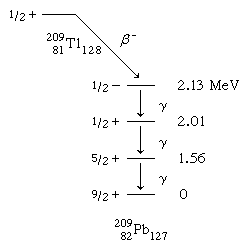that there are available g9/2d5/2, and s1/2 orbitals with which to explain the ground and first two excited states. Low-lying states associated with the i11/2 and j15/2 orbitals are known from nuclear-reaction studies, but they are not populated in the beta decay.

The 2.13-MeV state that receives the primary beta decay is not so simply interpreted as the other states. It is to be associated with the promotion of a neutron from the 3p1/2 orbital below the 126 shell closure. The density (number per MeV) of states increases rapidly above this excitation, and the interpretations become more complex and less certain.

By suitable refinements, the spherical-shell model can be extended further from the doubly magic region. Primarily, it is necessary to drop the approximation that nucleons move independently in orbitals and to invoke a residual force, mainly short-range and attractive, between the nucleons. The spherical-shell model augmented by residual interactions can explain and correlate around the magic regions a large amount of data on binding energies, spins, magnetic moments, and the spectra of excited states.

## The collective model

For nuclei more removed from the doubly magic regions, the spherical-shell model encounters difficulty in explaining the large observed electric quadrupole moments indicating cigar-shaped nuclei. For these nuclei a hybrid of liquid-drop and shell models, the collective model, has been proposed. (See the circular regions of Figure 2 for occurrence of cigar-shaped nuclei.)

Nucleons can interact with one another in a collective fashion to deform the nuclear shape to a cigar shape. Such large spheroidal distortions are usual for nuclei far from magic, notably with 150 ≲ A ≲ 190, and 224 ≲ A (the symbol < denotes less than, and ∼ means that the number is approximate). In these deformed regions the collective model prescribes that orbitals be computed in a cigar-shaped potential and that the relatively low-energy rotational excitations of the tumbling motion of the cigar shape be taken into account. The collective model has been highly successful in correlating and predicting nuclear properties in deformed regions. An example of a nuclear rotational band (a series of adjacent states) is provided by the decay of the isomer hafnium-180m, in Figure 3, through a cascade of gamma rays down the ground rotational band (see below Gamma transition for explanation of M2, E1, E2, and E3).decay of hafnium-180Figure 3: The decay scheme of hafnium-180m (see text).Encyclopædia Britannica, Inc.

There is a vast range of the rates of radioactive decay, from undetectably slow to unmeasurably short. Before considering the factors governing particular decay rates in detail, it seems appropriate to review the mathematical equations governing radioactive decay and the general methods of rate measurement in different ranges of half-life.

## Exponential-decay law

Radioactive decay occurs as a statistical exponential rate process. That is to say, the number of atoms likely to decay in a given infinitesimal time interval (dN/dt) is proportional to the number (N) of atoms present. The proportionality constant, symbolized by the Greek letter lambda, λ, is called the decay constant. Mathematically, this statement is expressed by the first-order differential equation,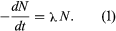This equation is readily integrated to give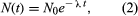in which N0 is the number of atoms present when time equals zero. From the above two equations it may be seen that a disintegration rate, as well as the number of parent nuclei, falls exponentially with time. An equivalent expression in terms of half-life t1⁄2 is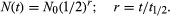It can readily be shown that the decay constant λ and half-life (t1⁄2) are related as follows: λ = loge2/t1⁄2 = 0.693/t1⁄2. The reciprocal of the decay constant λ is the mean life, symbolized by the Greek letter tau, τ.

For a radioactive nucleus such as potassium-40 that decays by more than one process (89 percent β−, 11 percent electron capture), the total decay constant is the sum of partial decay constants for each decay mode. (The partial half-life for a particular mode is the reciprocal of the partial decay constant times 0.693.) It is helpful to consider a radioactive chain in which the parent (generation 1) of decay constant λ1 decays into a radioactive daughter (generation 2) of decay constant λ2. The case in which none of the daughter isotope (2) is originally present yields an initial growth of daughter nuclei followed by its decay. The equation giving the number (N2) of daughter nuclei existing at time t in terms of the number N1(0) of parent nuclei present when time equals zero is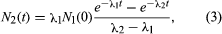in which e represents the logarithmic constant 2.71828.

The general equation for a chain of n generations with only the parent initially present (when time equals zero) is as follows: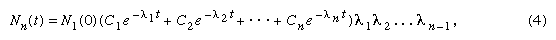in which e represents the logarithmic constant 2.71828.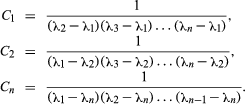These equations can readily be modified to the case of production of isotopes in the steady neutron flux of a reactor or in a star. In such cases, the chain of transformations might be mixed with some steps occurring by neutron capture and some by radioactive decay. The neutron-capture probability for a nucleus is expressed in terms of an effective cross-sectional area. If one imagines the nuclei replaced by spheres of the same cross-sectional area, the rate of reaction in a neutron flux would be given by the rate at which neutrons strike the spheres. The cross section is usually symbolized by the Greek letter sigma, σ, with the units of barns (10−24 cm2) or millibarns (10−3 b) or microbarns (10−6 b). Neutron flux is often symbolized by the letters nv (neutron density, n, or number per cubic centimetre, times average speed, v) and given in neutrons per square centimetre per second.

The modification of the transformation equations merely involves substituting the product nvσi in place of λi for any step involving neutron capture rather than radioactive decay. Reactor fluxes nv even higher than 1015 neutrons per square centimetre per second are available in several research reactors, but usual fluxes are somewhat lower by a factor of 1,000 or so. Tables of neutron-capture cross sections of the naturally occurring nuclei and some radioactive nuclei can be used for calculation of isotope production rates in reactors.

## Measurement of half-life

The measurement of half-lives of radioactivity in the range of seconds to a few years commonly involves measuring the intensity of radiation at successive times over a time range comparable to the half-life. The logarithm of the decay rate is plotted against time, and a straight line is fitted to the points. The time interval for this straight-line decay curve to fall by a factor of 2 is read from the graph as the half-life, by virtue of equations (1) and (2). If there is more than one activity present in the sample, the decay curve will not be a straight line over its entire length, but it should be resolvable graphically (or by more sophisticated statistical analysis) into sums and differences of straight-line exponential terms. The general equations (4) for chain decays show a time dependence given by sums and differences of exponential terms, though special modified equations are required in the unlikely case that two or more decay constants are identically equal.

For half-lives longer than several years it is often not feasible to measure accurately the decrease in counting rate over a reasonable length of time. In such cases, a measurement of specific activity may be resorted to; i.e., a carefully weighed amount of the radioactive isotope is taken for counting measurements to determine the disintegration rate, D. Then by equation (1) the decay constant λi may be calculated. Alternately, it may be possible to produce the activity of interest in such a way that the number of nuclei, N, is known, and again with a measurement of D equation (1) may be used. The number of nuclei, N, might be known from counting the decay of a parent activity or from knowledge of the production rate by a nuclear reaction in a reactor or accelerator beam.

Half-lives from 100 microseconds to one nanosecond are measured electronically in coincidence experiments. The radiation yielding the species of interest is detected to provide a start pulse for an electronic clock, and the radiation by which the species decays is detected in another device to provide a stop pulse. The distribution of these time intervals is plotted semi-logarithmically, as discussed for the decay-rate treatment, and the half-life is determined from the slope of the straight line.

Half-lives in the range of 100 microseconds to one second must often be determined by special techniques. For example, the activities produced may be deposited on rapidly rotating drums or moving tapes, with detectors positioned along the travel path. The activity may be produced so as to travel through a vacuum at a known velocity and the disintegration rate measured as a function of distance; however, this method usually applies to shorter half-lives in or beyond the range of the electronic circuit.

Species with half-lives shorter than the electronic measurement limit are not considered as separate radioactivities, and the various techniques of determining their half-lives will hence not be cited here.

Decay-rate considerations for various types of radioactivity are given here in the same order as listed above in Types of radioactivity.

# Alpha decay

Alpha decay, the emission of helium ions, exhibits sharp line spectra when spectroscopic measurements of the alpha-particle energies are made. For even–even alpha emitters the most intense alpha group or line is always that leading to the ground state of the daughter. Weaker lines of lower energy go to excited states, and there are frequently numerous lines observable.

The main decay group of even–even alpha emitters exhibits a highly regular dependence on the atomic numberZ, and the energy release, Qα. (Total alpha energy release, Qα, is equal to alpha-particle energy, Eα, plus daughter recoil energy needed for conservation of momentum; Erecoil = (mα/[mα + Md])Eα, with mα equal to the mass of the alpha particle and Md the mass of the daughter product.) As early as 1911 the German physicist Johannes Wilhelm Geiger, together with the British physicist John Mitchell Nuttall, noted the regularities of rates for even–even nuclei and proposed a remarkably successful equation for the decay constant, log λ = a + b log r, in which r is the range in air, b is a constant, and a is given different values for the different radioactive series. The decay constants of odd alpha emitters (odd A or odd Z or both) are not quite so regular and may be much smaller. The values of the constant b that were used by Geiger and Nuttall implied a roughly 90th-power dependence of λ on Qα. There is a tremendous range of known half-lives from the 2 × 1015 years of 144/60Nd (neodymium) with its 1.83-MeV alpha-particle energy (Eα) to the 0.3 microsecond of 212/84Po (polonium) with Eα = 8.78 MeV.

The theoretical basis for the Geiger–Nuttall empirical rate law remained unknown until the formulation of wave mechanics. A dramatic early success of wave mechanics was the quantitative theory of alpha-decay rates. One curious feature of wave mechanics is that particles may have a nonvanishing probability of being in regions of negative kinetic energy. In classical mechanics a ball that is tossed to roll up a hill will slow down until its gravitational potential energy equals its total energy, and then it will roll back toward its starting point. In quantum mechanics the ball has a certain probability of tunneling through the hill and popping out on the other side. For objects large enough to be visible to the eye, the probability of tunneling through energetically forbidden regions is unobservably small. For submicroscopic objects such as alpha particles, nucleons, or electrons, however, quantum mechanical tunneling can be an important process—as in alpha decay.

The logarithm of tunneling probability on a single collision with an energy barrier of height B and thickness D is a negative number proportional to thickness D, to the square root of the product of B and particle mass m. The size of the proportionality constant will depend on the shape of the barrier and will depend inversely on Planck’s constant h.

In the case of alpha decay, the electrostatic repulsive potential between alpha particle and nucleus generates an energetically forbidden region, or potential barrier, from the nuclear radius out to several times this distance. The maximum height (B) of this alpha barrier is given approximately by the expression B = 2Ze2/R, in which Z is the charge of the daughter nucleus, e is the elementary charge in electrostatic units, and R is the nuclear radius. Numerically, B is roughly equal to 2Z/A1⁄3, with A the mass number and B in energy units of MeV. Thus, although the height of the potential barrier for 212/84Po decay is nearly 28 MeV, the total energy released is Qα = 8.95 MeV. The thickness of the barrier (i.e., distance of the alpha particle from the centre of the nucleus at the moment of recoil) is about twice the nuclear radius of 8.8 × 10−13 centimetre. The tunneling calculation for the transition probability (P) through the barrier gives approximately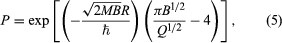in which M is the mass of the alpha particle and ℏ is Planck’s constant h divided by 2π. By making simple assumptions about the frequency of the alpha particle striking the barrier, the penetration formula (5) can be used to calculate an effective nuclear radius for alpha decay. This method was one of the early ways of estimating nuclear sizes. In more sophisticated modern techniques the radius value is taken from other experiments, and alpha-decay data and penetrabilities are used to calculate the frequency factor.

The form of equation (5) suggests the correlation of decay rates by an empirical expression relating the half-life (t1⁄2) of decay in seconds to the release energy (Qα) in MeV: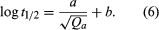Values of the constants a and b that give best fits to experimental rates of even–even nuclei with neutron number greater than 126 are given in the table. The nuclei with 126 or fewer neutrons decay more slowly than the heavier nuclei, and constants a and b must be readjusted to fit their decay rates.

The alpha-decay rates to excited states of even–even nuclei and to ground and excited states of nuclei with odd numbers of neutrons, protons, or both may exhibit retardations from equation (6) rates ranging to factors of thousands or more. The factor by which the rate is slower than the rate formula (6) is the hindrance factor. The existence of uranium-235 in nature rests on the fact that alpha decay to the ground and low excited states exhibits hindrance factors of over 1,000. Thus the uranium-235 half-life is lengthened to 7 × 108 years, a time barely long enough compared to the age of the elements in the solar system for uranium-235 to exist in nature today.

The alpha hindrance factors are fairly well understood in terms of the orbital motion of the individual protons and neutrons that make up the emitted alpha particle. The alpha-emitting nuclei heavier than radium are considered to be cigar-shaped, and alpha hindrance factor data have been used to infer the most probable zones of emission on the nuclear surface—whether polar, equatorial, or intermediate latitudes.

# Beta decay

The processes separately introduced at the beginning of this section as beta-minus decay, beta-plus decay, and orbital electron capture can be appropriately treated together. They all are processes whereby neutrons and protons may transform to one another by weak interaction. In striking contrast to alpha decay, the electrons (minus or plus charged) emitted in beta-minus and beta-plus decay do not exhibit sharp, discrete energy spectra but have distributions of electron energies ranging from zero up to the maximum energy release, Qβ . Furthermore, measurements of heat released by beta emitters (most radiation stopped in surrounding material is converted into heat energy) show a substantial fraction of the energy, Qβ , is missing. These observations, along with other considerations involving the spins or angular momenta of nuclei and electrons, led Wolfgang Pauli to postulate the simultaneous emission of the neutrino (1931). The neutrino, as a light and uncharged particle with nearly no interaction with matter, was supposed to carry off the missing heat energy. Today, neutrino theory is well accepted with the elaboration that there are six kinds of neutrinos, the electron neutrino, mu neutrino, and tau neutrino and corresponding antineutrinos of each. The electron neutrinos are involved in nuclear beta-decay transformations, the mu neutrinos are encountered in decay of muons to electrons, and the tau neutrinos are produced when a massive lepton called a tau breaks down.

Although in general the more energetic the beta decay the shorter is its half-life, the rate relationships do not show the clear regularities of the alpha-decay dependence on energy and atomic number.

The first quantitative rate theory of beta decay was given by Enrico Fermi in 1934, and the essentials of this theory form the basis of modern theory. As an example, in the simplest beta-decay process, a free neutron decays into a proton, a negative electron, and an antineutrino: n → p + e + ν. The weak interaction responsible for this process, in which there is a change of species (n to p) by a nucleon with creation of electron and antineutrino, is characterized in Fermi theory by a universal constantg. The sharing of energy between electron and antineutrino is governed by statistical probability laws giving a probability factor for each particle proportional to the square of its linear momentum (defined by mass times velocity for speeds much less than the speed of light and by a more complicated, relativistic relation for faster speeds). The overall probability law from Fermi theory gives the probability per unit time per unit electron energy interval, P(W), as follows: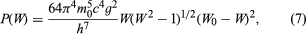in which W is the electron energy in relativistic units (W = 1 + E/m0c2) and W0 is the maximum (W0 = 1 + Qβ/m0c2), m0 the rest mass of the electron, c the speed of light, and h Planck’s constant. This rate law expresses the neutron beta-decay spectrum in good agreement with experiment, the spectrum falling to zero at lowest energies by the factor W and falling to zero at the maximum energy by virtue of the factor (W0 − W)2.

In Fermi’s original formulation, the spins of an emitted beta and neutrino are opposing and so cancel to zero. Later work showed that neutron beta decay partly proceeds with the 1/2 ℏ spins of beta and neutrino adding to one unit of ℏ. The former process is known as Fermi decay (F) and the latter Gamow–Teller (GT) decay, after George Gamow and Edward Teller, the physicists who first proposed it. The interaction constants are determined to be in the ratio gGT2/gF2 = 1.4. Thus, g2 in equation (7) should be replaced by (gF2 + gGT2).

The scientific world was shaken in 1957 by the measurement in beta decay of maximum violation of the law of conservation of parity. The meaning of this nonconservation in the case of neutron beta decay considered above is that the preferred direction of electron emission is opposite to the direction of the neutron spin. By means of a magnetic field and low temperature it is possible to cause neutrons in cobalt-60 and other nuclei, or free neutrons, to have their spins set preferentially in the up direction perpendicular to the plane of the coil generating the magnetic field. The fact that beta decay prefers the down direction for spin means that the reflection of the experiment as seen in a mirror parallel to the coil represents an unphysical situation: conservation of parity, obeyed by most physical processes, demands that experiments with positions reversed by mirror reflection should also occur. Further consequences of parity violation in beta decay are that spins of emitted neutrinos and electrons are directed along the direction of flight, totally so for neutrinos and partially so by the ratio of electron speed to the speed of light for electrons.

The overall half-life for beta decay of the free neutron, measured as 12 minutes, may be related to the interaction constants g2 (equal to gF2 + gGT2) by integrating (summing) probability expression (7) over all possible electron energies from zero to the maximum. The result for the decay constant isin which W0 is the maximum beta-particle energy in relativistic units (W0 = 1 + Qβ/m0c2), with m0 the rest mass of the electron, c the speed of light, and h Planck’s constant. The best g value from decay rates is approximately 10−49 erg per cubic centimetre. As may be noted from equation (8), there is a limiting fifth-power energy dependence for highest decay energies.

In the case of a decaying neutron not free but bound within a nucleus, the above formulas must be modified. First, as the nuclear charge Z increases, the relative probability of low-energy electron emission increases by virtue of the coulombic attraction. For positron emission, which is energetically impossible for free protons but can occur for bound protons in proton-rich nuclei, the nuclear coulomb charge suppresses lower energy positrons from the shape given by equation (7). This equation can be corrected by a factor F(Z,W) depending on the daughter atomic number Z and electron energy W. The factor can be calculated quantum mechanically. The coulomb charge also affects the overall rate expression (8) such that it can no longer be expressed as an algebraic function, but tables are available for analysis of beta decay rates. The rates are analyzed in terms of a function f(Z,Qβ) calculated by integration of equation (7) with correction factor F(Z,W).

Approximate expressions for the f functions usable for decay energies Q between 0.1 MeV and 10 MeV, in which Q is measured in MeV, and Z is the atomic number of the daughter nucleus, are as follows (the symbol ≈ means approximately equal to):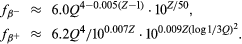For electron capture, a much weaker dependence on energy is found: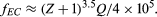The basic beta decay rate expression obeyed by the class of so-called superallowed transitions, including decay of the neutron and several light nuclei is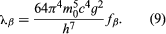Like the ground-to-ground alpha transitions of even–even nuclei, the superallowed beta transitions obey the basic rate law, but most beta transitions go much more slowly. The extra retardation is explained in terms of mismatched orbitals of neutrons and protons involved in the transition. For the superallowed transitions the orbitals in initial and final states are almost the same. Most of them occur between mirror nuclei, with one more or less neutron than protons; i.e., beta-minus decay of hydrogen-3, electron capture of beryllium-7 and positron emission of carbon-11, oxygen-15, neon-19, . . . titanium-43.

The nuclear retardation of beta decay rates below those of the superallowed class may be expressed in a fundamental way by multiplying the right side of equation (9) by the square of a nuclear matrix element (a quantity of quantum mechanics), which may range from unity down to zero depending on the degree of mismatch of initial and final nuclear states of internal motion. A more usual way of expressing the nuclear factor of the beta rate is the log ft value, in which f refers to the function f(Z,Qβ). Because the half-life is inversely proportional to the decay constant λ, the product fβt1⁄2 will be a measure of (inversely proportional to) the square of the nuclear matrix element. For the log ft value, the beta half-life is taken in seconds, and the ordinary logarithm to the base 10 is used. The superallowed transitions have log ft values in the range of 3 to 3.5. Beta log ft values are known up to as large as ∼ 23 in the case of indium-115. There is some correlation of log ft values with spin changes between parent and daughter nucleons, the indium-115 decay involving a spin change of four, whereas the superallowed transitions all have spin changes of zero or one.

## Gamma transition

The nuclear gamma transitions belong to the large class of electromagnetic transitions encompassing radio-frequency emission by antennas or rotating molecules, infrared emission by vibrating molecules or hot filaments, visible light, ultraviolet light, and X-ray emission by electronic jumps in atoms or molecules. The usual relations apply for connecting frequency ν, wavelength λ, and photon quantum energy E with speed of light c and Planck’s constant h; namely, λ = c/ν and E = hv. It is sometimes necessary to consider the momentum (p) of the photon given by p = E/c.

Classically, radiation accompanies any acceleration of electric charge. Quantum mechanically there is a probability of photon emission from higher to lower energy nuclear states, in which the internal state of motion involves acceleration of charge in the transition. Therefore, purely neutron orbital acceleration would carry no radiative contribution.

Transition rates are usually compared to the single-proton theoretical rate, or Weisskopf formula, named after the American physicist Victor Frederick Weisskopf, who developed it. The table gives the theoretical reference rate formulas in their dependence on nuclear mass number A and gamma-ray energy Eγ (in MeV).

It is seen for the illustrative case of gamma energy 0.1 MeV and mass number 125 that there occurs an additional factor of 107 retardation with each higher multipole order. For a given multipole, magnetic radiation should be a factor of 100 or so slower than electric. These rate factors ensure that nuclear gamma transitions are nearly purely one multipole, the lowest permitted by the nuclear spin change. There are many exceptions, however; mixed M1–E2 transitions are common, because E2 transitions are often much faster than the Weisskopf formula gives and M1 transitions are generally slower. All E1 transitions encountered in radioactivity are much slower than the Weisskopf formula. The other higher multipolarities show some scatter in rates, ranging from agreement to considerable retardation. In most cases the retardations are well understood in terms of nuclear model calculations.

Though not literally a gamma transition, electric monopole (E0) transitions may appropriately be mentioned here. These may occur when there is no angular momentum change between initial and final nuclear states and no parity change. For spin-zero to spin-zero transitions, single gamma emission is strictly forbidden. The electric monopole transition occurs largely by the ejection of electrons from the orbital cloud in heavier elements and by positron–electron pair creation in the lighter elements.

## In medicine

Radioisotopes have found extensive use in diagnosis and therapy, and this has given rise to a rapidly growing field called nuclear medicine. These radioactive isotopes have proven particularly effective as tracers in certain diagnostic procedures. As radioisotopes are identical chemically with stable isotopes of the same element, they can take the place of the latter in physiological processes. Moreover, because of their radioactivity, they can be readily traced even in minute quantities with such detection devices as gamma-ray spectrometers and proportional counters. Though many radioisotopes are used as tracers, iodine-131, phosphorus-32, and technetium-99m are among the most important. Physicians employ iodine-131 to determine cardiac outputplasma volume, and fat metabolism and particularly to measure the activity of the thyroid gland where this isotope accumulates. Phosphorus-32 is useful in the identification of malignant tumours because cancerous cells tend to accumulate phosphates more than normal cells do. Technetium-99m, used with radiographic scanning devices, is valuable for studying the anatomic structure of organs.

Such radioisotopes as cobalt-60 and cesium-137 are widely used to treat cancer. They can be administered selectively to malignant tumours and so minimize damage to adjacent healthy tissue.

## In science

Research in the Earth sciences has benefited greatly from the use of radiometric-dating techniques, which are based on the principle that a particular radioisotope (radioactive parent) in geologic material decays at a constant known rate to daughter isotopes. Using such techniques, investigators have been able to determine the ages of various rocks and rock formations and thereby quantify the geologic time scale (see geochronology: Absolute dating). A special application of this type of radioactivity age method, carbon-14 dating, has proved especially useful to physical anthropologists and archaeologists. It has helped them to better determine the chronological sequence of past events by enabling them to date more accurately fossils and artifacts from 500 to 50,000 years old.

Radioisotopic tracers are employed in environmental studies, as, for instance, those of water pollution in rivers and lakes and of air pollution by smokestack effluents. They also have been used to measure deep-water currents in oceans and snow-water content in watersheds. Researchers in the biological sciences, too, have made use of radioactive tracers to study complex processes. For example, thousands of plant metabolic studies have been conducted on amino acids and compounds of sulfur, phosphorus, and nitrogen.John O. RasmussenEllis P. Steinberg

Publicité

## Publié par zeggaoui el mostafa

professeur agrégé de physique et chimie ; militant pour l'apprentissage et la diffusion de la culture scientifique en général , physique chimique essentiellement , ainsi que , je contribue à développer un projet d'apprentissage scientifique à distance , en aidant les élèves en publiant un ensemble de leçons et des exercices de physique chimique , du cycle secondaire qualifiant marocain

## 3 commentaires sur « Fission nucléaire et fusion nucléaire : quelle différence ? | Dossier Radioactivity »

1.zeggaoui el mostafa dit :

Pour des raisons techniques, la fission est relativement considérée facile par rapport à la fusion. Mais, l’avenir est en faveur de la fusion, car elle utilise un combustible existant en grande quantité qu’est l’hydrogène

J’aime